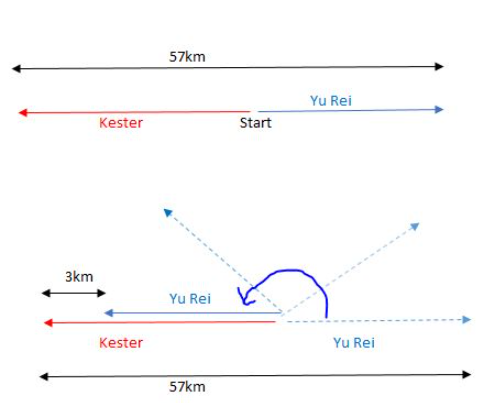# Question

In 2 hours,

1. Kester and Yu Rei cycle 57 km in total.
2. Kester cycles 3 km more.

Yu Rei ——- 1u

Kester ——- 1u + 3

1u + 1u + 3 = 2u + 3 ——- 57

1u ——- (57 – 3)/2 = 27Hi Chloecube, hope the picture can help you in visualising the directions, sum/difference in distances and units. Yu Rei travelled 1 unit distance.

Kester and Yu Rei start cycling at the same time from the same place.

If they cycle in the opposite direction, they will be 57 km apart after 2 hours ——- Kester and Yu Rei cycle a total distance of 57 km after 2 hours.

If they cycle in the same direction, Kester will be 3 km ahead of Yu Rei after two hours ——- Yu Rei cycles 1u km and Kester cycles (1u + 3) km after 2 h.

Could you understand now?

0 Replies 1 Like

57 – 3 = 54
54/2 = 27 (distance travelled by Yu Rei in 2 h)
27 + 3 = 30 (distance travelled by Kester in 2 h)
30/2 = 15

Ans : 15 km/h.

In 2 hours,

1. Kester and Yu Rei cycle 57 km in total.
2. Kester cycles 3 km more.

Yu Rei ——- 1u

Kester ——- 1u + 3

1u + 1u + 3 = 2u + 3 ——- 57

1u ——- (57 – 3)/2 = 27Hi Chloecube, hope the picture can help you in visualising the directions, sum/difference in distances and units. Yu Rei travelled 1 unit distance.

Kester and Yu Rei start cycling at the same time from the same place.

If they cycle in the opposite direction, they will be 57 km apart after 2 hours ——- Kester and Yu Rei cycle a total distance of 57 km after 2 hours.

If they cycle in the same direction, Kester will be 3 km ahead of Yu Rei after two hours ——- Yu Rei cycles 1u km and Kester cycles (1u + 3) km after 2 h.

Could you understand now?

0 Replies 1 Like

Maybe if you look at cycling opposite direction as the same as the total distance of Kester and Yurei, it should be easier to understand.

For 2 hours,
Yurei traveled 1u
Kester traveled 1u+3

If they traveled opposite direction, is the same as the total distance.
57km = Yurei + Kester = 1u + 1u + 3
57km =2u + 3
u = (57 – 3 )/ 2 = 27

In 2 hours, Kester traveled = 1u+3 = 27 + 3 =30km
Kester’s speed = 30/2 – 15km/hr ###

0 Replies 1 Like

In 2 hours, K and Y cycled 57km altogether and K cycled 3km more than Y.

Hence, K cycled 30km and Y cycled 27km.

K average speed is 30 km in 2 hours = 15km/h

0 Replies 0 Likes